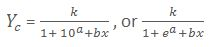# Calculating Growth Rate in Time Series

By a time series we mean a series of value of a variable, the values of which vary according to the passage of time. In such type of variables, the time factor plays an important role in affecting the variable to a marked extent. The examples of such series may be cited as under:

(i)  A series relating to consumption, production, or prices of certain goods.

(ii)  A series relating to purchase, sales, profits, or losses of a certain business concern.

(iii) A series relating to agricultural of industrial production, investments, foreign exchange reserves, population, crimes, national income or imports and exports of a company.

(iv) A series relating to bank deposits, bank clearings, prices of shares, or dividend rates of a certain company.

(v)  A series relating to temperature, rainfall, or yield of a particular area.

Time series cited as above has been defined variously by various authors. Some important ones among those are quoted as under:

• According to Croxton and Cowden, “A time series consists of data arrayed chronologically”.
• According to Kenny and Keeping, “A set of data depending on the time is called time series”.
• In the words of Ya-Lun-Chou, “A time series may be defined as a collection of readings belonging to different time periods, of some economic variables such as production of steel, per capita income, gross national products, price of tobacco, or index of industrial production.”

### Characteristics

From all the above definitions, the essential characteristics of a time series may be derived as under:

(i)  It consists of a set of values of a variable with reference to the time of their occurrence. As such, a series to constitute a time series must exhibit the data in two columns at least viz: (i) time columns, and (ii) value column.

(ii) It consists of the data quite for a long period, say, 7,10,20, or 30 periods of years, months, weeks or days which may be reasonable for the problem in issue.

(iii) It consists of equal time gaps between the various values of a variable, viz.: 1999, 2000, 2001, 2002, and 2003 etc. or January, February, March, April etc. This means that the data must have been obtained in equal order of chronology.

(iv) It represents a variable the values of which are affected by the time factor. For example, the price of woolen products goes put during the winter season and come down during the summer season. As such, the gap, if any, in the data must be capable of being interpolated or extrapolated with reference to the effect of the time factor.

The growth curves are some special type of curves which are plotted on graph papers for analyzing and estimating the trend values in the business and economic phenomena. Where initially, the growth rate is very slow but gradually it picks up at a faster rate till it reaches a point of stagnation, or saturation. Such situations are quite common in business fields where new products are introduced for marketing. In such a situation, when a new product say the book, “Statistical Methods” is introduced into the market, the growth rate of its sale is quite slow, and if the product proves to be worthwhile, the growth rate of its begins to decline. As such, a curve, representing this type of phenomena continues to grow more and more slowly approaching the upper limit, but never reaching the same. It takes the shape of an elongated f which indicates the pattern of growth in terms of the actual amount as small in the early years increasingly greater in the middle Years, and large and stabilized in the later years. But when such curves are plotted on a semi logarithmic chart, they show a growth at rapidly increasing rates in the earlier periods and at a declining ratio in the later periods of the series.

There are different types of growth curves used in different fields of business and economics, but the most popular among them are the following twos which have come into being since 1920:

1. Gompertz Growth Curve.
2. Logistic or Pearl-Read Growth Curve.

A brief introduction to these curves is given as below:

Gompertz Growth Curve. The fundamental trend equation of this curve is given by

Yc = Kabx

When put to the logarithmic form, the above equation is modified as under:

Ye = Al of [log K + bX log a]

Where, k represents the constants of the highest point, an intercept of y i.e. the trend value of the origin of X, and b the slope of the line i.e. the rate of growth.

This curve is given by the following model

1/yc = k + ab×

The above equation may also be used in the alternative form as under:

It may be noted that the Logistic curve is nothing but a modified exponential curve in terms of the reciprocal of the y variable. This curve, however, is very popular in the demographic studies and in many business and economic analysis.### 1 Comment »

error: Content is protected !!